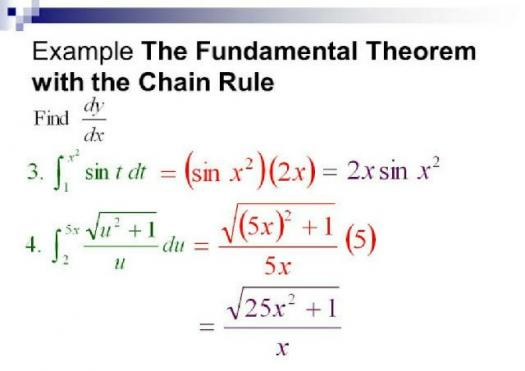# AP Calculus: The Fundamental Theorem Of Calculus! Practice Test!

10 Questions | Total Attempts: 209SettingsAdvanced Placement Calculus is not for everybody or do you think it is? Well, Fundamental theorem under AP Calculus basically deals with function, integration and derivation and while many see it as hard but to crack, we think its a fun topic for a start and would really advise you to take this quick test quiz on it just to boost your knowledge of the topic. Enjoy!

• 1.
What is the inverse function of f(x) = x/x+1?
• A.

F^-1 (x) = –x/x–1

• B.

F²(x) = x/x-2²

• C.

F^-1 (x) = x/x + 1

• D.

F^-1 (x) = x-1/ x + 2

• 2.
Suppose the operation 0 is defined by the relation p 0 q = max {p, q}. Evaluate 304
• A.

41

• B.

4

• C.

40

• D.

400

• 3.
What is the derivative of y = X^7 + cosX + sinX + √x + 3√x²?
• A.

7x6 - sinX + cosX + 1/ 3√x + 2/ 3√x

• B.

7x²- cosX + 1/2x + 3/ 2√x

• C.

7x³ - sinX - 12√2x - cos X

• D.

7x - sinX + cosX - 2√x²

• 4.
What is the differential coefficient of y = 2x/4 + x?
• A.

2/ (8 + x)²

• B.

4/ (1 - x)³

• C.

8/ (4 + x)²

• D.

8/ 4 + x²

• 5.
Find dy/dx of sinX + x / x² + cosX
• A.

X - sinX + cosX + √x + 3√x / x²- cosX

• B.

X²- cosX + 1/2x + 3/ 2√x/ (2x²- sinX)

• C.

X³ - sinX - √2x - cos X / (x² + cosX)²

• D.

(x² + 1) cosX - XsinX - x² + 1 / (x² + cosX)

• 6.
U = ∅sin∅/ 2∅² + 1 find dy/dx
• A.

(2∅² + 1) ∅cos∅ + (1 - 2∅²) sin∅ / (2∅²+ 1)²

• B.

(3∅² + 1) ∅cos∅ + (1 - 2∅³) sin∅ / (2∅+ 1)

• C.

(2∅² + 1) ∅cos∅ + (1 - 2∅²) sin∅ / (2∅²+ 1)²

• D.

(∅² + 1) cos∅ + (1 - ∅²) sin∅ / (2∅²+ 1)²

• 7.
Find dy/dx of y = sin²X
• A.

2sinX cosX

• B.

SinX cosX

• C.

Sin²X cos⁴X

• D.

2sinX 2cosX

• 8.
Differentiating y = 1/√x + 2 with respect to x gives:
• A.

1√x³ + 2

• B.

− 1/2√x³

• C.

2 - 1/2√x³

• D.

2/√x³

• 9.
Given f(t) = 3t⁴ − 2, f(t) is equal to:
• A.

12t³ − 2

• B.

3/4 t5 − 2t + c

• C.

12t³

• D.

3t5 − 2

• 10.
An alternating voltage is given by v = 10 sin 300t volts, where t is the time in seconds. The rate of change of voltage when t = 0.01 s is:
• A.

-2996V/s

• B.

157V/s

• C.

-2970V/s

• D.

0.523V/s

Related TopicsBack to top